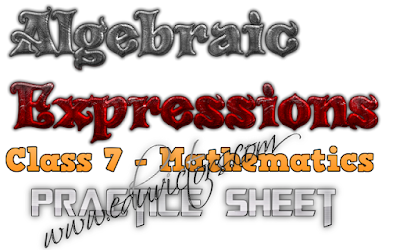## Class 7 - Maths - Algebraic Expressions - Practice Sheet

Q1. Classify as monomial, binomial and trinomial.

(a) x - x² + x³
(b) -26ab
(c) -7p²q -7pq²
(d) -27a² -125b²
(e) 2978

Q2. Form algebraic expressions from the following statements.

(a) Sum of the terms 5x and (-9y)
(b) Sum of the squares of 4 and x
(c) Product of a and b added to the sum of y and 20.
(d) Two times number 9 is subtracted from the product of y and z.

Q3. Simplify and write:

(a) 7x³ -6xy -18x² + yx
(b) 16xy - 3(x - xy)
(c) 9x²y + yx² + 8xy - 9yx² + 3xy
(d) (z + 3) - (z - 3) + (z - 6)

Q4. Find the value of each of the following expression if x = 2, y = 3 and z = 1

(a) x – y + z
(b) x² + y² + z²
(c) 2x + y² – z² + 3

Q5. Subtract the sum of 2x +3y +5z and -3x – y – 2z from the sum of 3x – 2y + z and 2x + 2y +3z

Q6. Form the expressions from the following statements:

(a) The sum of a number and 12 is 27.
(b) Half of a number is 19.
(c) Three times a number plus 10 is 97.
(d) Twice the sum of a number and 5 is 12.
(e) Ten less than twice the number is 86.

Q7. Check the solution of the following equations:

(a) 3x - 14 = 16, x = 10
(b) 4(2y + 1 ) = 36, y = 6
(c) 12a + 4 = 40, a = 2
(d) 3(2x - 1) + 5 = 14, x = 2#### 2 comments:

1.Very typical and good questions

2.We love to hear your thoughts about this post!